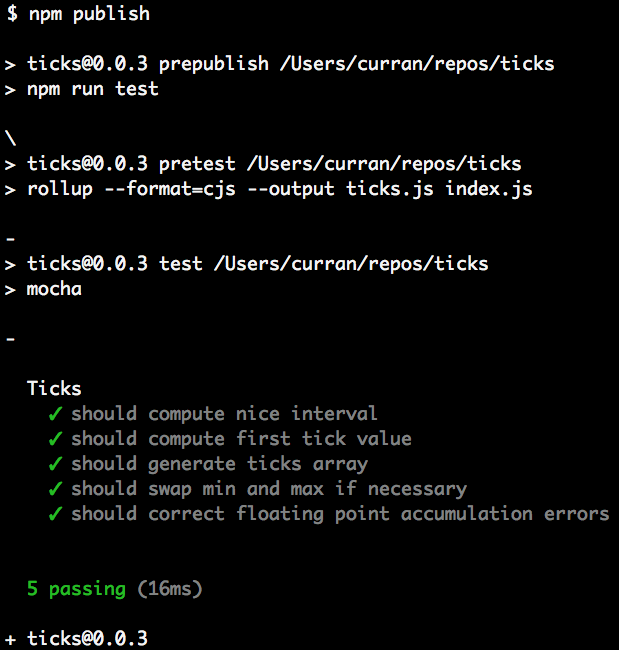Noble Pug Mullet

# npm

Need private packages and team management tools?Check out npm Orgs. »

## ticks

0.0.4 • Public • Published

# Ticks

A utility for choosing nice tick marks or histogram intervals.

## API

`ticks(min, max, n)`

• `min` The minimum value of the interval.
• `max` The maximum value of the interval.
• `n` The approximate number of desired ticks.

Returns an array of "ticks", numbers that are suitable to use as tick mark values. The first tick will be less than or equal to `min`, and the last tick will be greater than or equal to `max`.

## Usage

Install via NPM:

`npm install -S ticks`

Example use:

## API

# ticks(min, max, numTicks [,tight])

Computes and returns approximately `numTicks` ticks spaced nicely that include the intervaj [min, max].

If `tight` is specified, then the first tick is the min, and the last tick is the max. The The Graphics Gems chapter "Nice Numbers for Graph Labels" by Paul S. Heckbert introduces the notion of "loose" and "tight" tick marks.

## Algorithm

The algorithm for computing ticks is based on the idea of a "nice interval". Nice intervals can be expressed as `(base * 10^exp)`, where `exp` is some integer exponent, and `base` is either 1, 2, or 5. Examples of nice intervals are 0.1, 0.5, 10, 20, 5, 2, and 500.

The Ticks algorithm computes the exponent of the raw interval, by `Math.log10((max - min) / n)`, then computes both the floor and ceiling of this value, which are candidate exponents for use in generating nice intervals. The algorithm then tries all 6 possible combinations of the two candidate exponents with the possible bases (1, 2, and 5) to generate a set of candidate nice intervals. From the generated set of nice intervals, the one that is closest to the raw interval (`(max - min) / n`) is chosen.

## Related Work

The seminal approach for generating tick marks appeared in "Nice Numbers for Graph Labels" by Paul S. Heckbert in the book "Graphics Gems", originally published in 1990.

Subsequently, there have been a variety of new takes on the problem. This research paper presents an approach for this that takes more factors into account, including the actual size of label text: An Extension of Wilkinson’s Algorithm for Positioning Tick Labels on Axes, by Justin Talbot, Sharon Lin, and Pat Hanrahan, and also surveys other algorithms.

Also, a similar algorithm is implemented in D3.scale.linear.ticks.

## Development Tooling

This module is published as an NPM package. The module itself is authored using ES6 module syntax in `index.js`, which is declared as the `jsnext:main` entry point in `package.json` so it can be included in Rollup-based builds. The module is converted to CommonJS format in the `prepublish` script, which runs the `pretest` and `test` scripts`, making the built file`ticks.js`available in the NPM package. The built file is excluded from the Git repository via the`.gitignore`file. Usually NPM ignores files in`.gitignore`, so to cause NPM to include`ticks.js`, an [empty`.npmignore` file was added.

Here's what it looks like when `npm publish` is run:During development you can run `npm test` to run the Rollup build and run the unit tests. The Mocha unit tests are written in ES5, and consume the CommonJS package generated by Rollup via Node.js.

Curran Kelleher June 2015

## Keywords

### install

`npm i ticks`

7

0.0.4

MIT

github.com

github